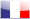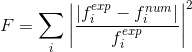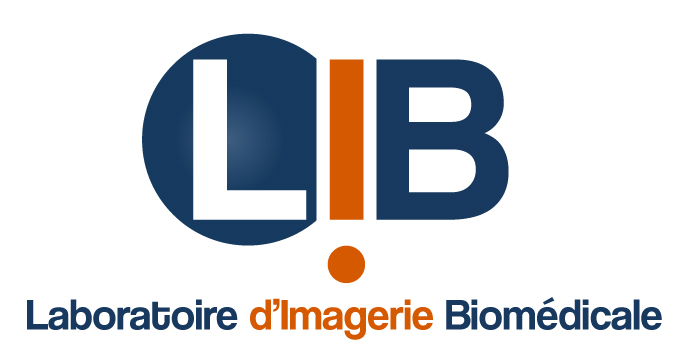Version française

### Resonant Ultrasound Spectroscopy (RUS)

The Resonant Ultrasound Spectroscopy is an experimental/numerical method. It aims to characterize the elastic tensor of a material sample. At the opposite of traditional elastic characterisation method (such as tensile test), the RUS method aims to determine the full tensor with one and only experiment.

RUS consists in measuring the frequency response of a material sample in order to obtain the eigenfrequencies and then match this set of frequencies with numerically calculated eigenfrequencies. Here it is the Finite Element Method (FEM) that is used to calculate those frequencies. So it is by minimising the relative error between the two set of frequencies and resolving an inverse problem that we get the elastic moduli of the sample. We are actually minimising a cost function F defined as the sum of the squared relative errors between experimental and numerical frequencies :The RUS method is schemed in the following figure :

The RUS method was invented in the early 1990's. At this time the numerically calculated eigenfrequencies was obtained with the Rayleigh-Ritz method which is only computable for simple shaped samples such as cylinders or cuboid. In this study we aim to implement this method using the finite element method to calculate the numerical frequencies in order to apply this method to arbitrarily shaped samples. The anisotropy of the material can also be implement in FEM.

We worked on the validation of the RUS method using FEM and the Levenberg-Marquart algorithm to solve the minimisation process. The major interest of the algorithm lies in the fact that it smartly combines two well known minimising algorithm : the gradient method and the Gauss-Newton algorithm. The relative influence of the two scheme is given by a parameter that first promote the gradient method and the Gauss-Newton method. This way, the algorithm go fast in the direction of the minimum and the linearise the cost function to find precisely the minimum point.

This algorithm is implemented in the optimisation toolbox of Matlab. That is why, for all this study we created a Matlab script suite that launch the mesh generation with GMSH, that launch the FEM calculation in Code_Aster, get back the results from a text file and calculate the cost function.

A synthesis of the script suite created during this study and who it is used to make the RUS method work is shown in the following figure :

In order to validate or calculation chain we apply RUS for a cylinder of copper, an isotropic material. Also, it has a very low damping so we will suppose equal the resonant frequencies and the eigenfrequencies of the sample. we can then calculate our cost function by calculating the relative error between the experimental resonant frequencies and REM calculated eigenfrequencies.

In order to be able to verify the results of the minimisation process we calculate a map of the cost function depending on C11 and C44, the two components of the elastic tensor for an isotropic material. The resulting map is presented in the figure below. An example of minimisation is also shown in this figure :

In this example the final point of iteration is very close the minimum of the map. This process took ten minutes using 2,5 GB of RAM with a 2,4 GHz single core processor.

During those first works we have developed a software suite allowing the RUS method using FEM for the numerical calculation of eigenfrequencies of the sample. All the steps explain before are controlled in Matlab and the minimisation process runs well in the case of isotropic material

We believe that this study can easily be upgraded to manage anisotropic materials by uprising the number of variables of the minimised function. Those variables are the components of the elastic tensor.

The results of this project are resumed in those documents :

Tutoring : Quentin GRIMAL. Laboratory :